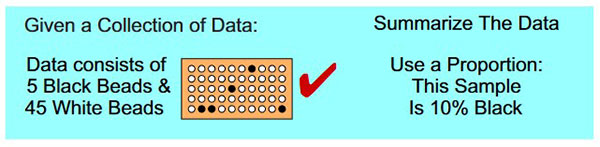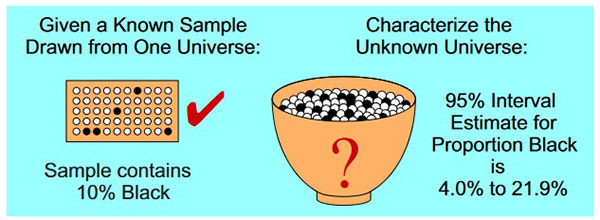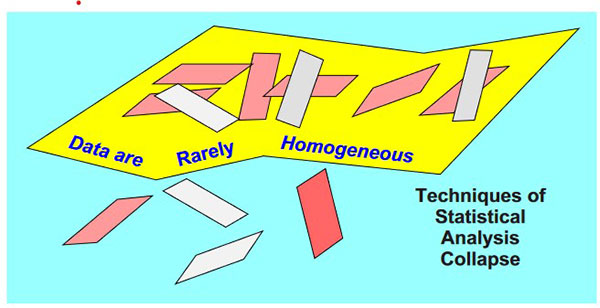#Quality Digest

Featured Product
This Week in Quality Digest Live
Statistics Features
Donald J. Wheeler
What does this ratio tell us?
Harish Jose
Any statistical statement we make should reflect our lack of knowledge
Donald J. Wheeler
How to avoid some pitfalls
Kari Miller
CAPA systems require continuous management, effectiveness checks, and support
Donald J. Wheeler
What happens when the measurement increment gets too large?
Statistics News
How to use Minitab statistical functions to improve business processes
New capability delivers deeper productivity insights to help manufacturers meet labor challenges
Day and a half workshop to learn, retain, and transfer GD&T knowledge across an organization
Elsmar Cove is a leading forum for quality and standards compliance
InfinityQS’ quality solutions have helped cold food and beverage manufacturers around the world optimize quality and safety
User friendly graphical user interface makes the R-based statistical engine easily accessible to anyone
Collect measurements, visual defect information, simple Go/No-Go situations from any online device
Good quality is adding an average of 11 percent to organizations’ revenue growth
Ability to subscribe with single-user minimum, floating license, and no long-term commitmentBio

Statistics

## The Secret of Data Analysis

### What they forgot to tell you in your statistics class

Published: Monday, December 5, 2022 - 13:03

There are four major questions in statistics. These can be listed under the headings of description, probability, inference, and homogeneity. An appreciation of the relationships between these four areas is essential for successful data analysis. This column outlines these relationships and provides a guide for data analyses.

### The description question

Given a collection of numbers, what arithmetic functions will summarize the information contained in those numbers in some meaningful way?

To be effective, a descriptive statistic has to make sense—it has to distill some essential characteristic of the data into a value that is both appropriate and understandable. In every case, this distillation takes on the form of some arithmetic operation:

Data + Arithmetic = Statistic

As soon as we have said this, it becomes apparent that the justification for computing any given statistic must come from the nature of the data themselves. The meaning of any statistic depends upon the context for the data, while the appropriateness of any statistic depends on the use we intend to make of that statistic.Figure 1: The problem of description

This means that before we compute the simplest average, range, or proportion, it has to make sense to do so. If the data are a meaningless collection of values, then the summary statistics will also be meaningless—arithmetic cannot magically create meaning out of nonsense.

Thus, we have to know the context for the data before we can select appropriate summary statistics. Once we have our descriptive statistics, we can then use them in answering the questions in the other three areas of statistics.

### The probability question

Given a known universe, what can we say about samples drawn from this universe?

Here we enter the world of deductive logic, of the enumeration of possible outcomes, and of probability models. For simplicity we usually begin with a universe that consists of a bowl filled with known numbers of black and white beads. We then consider the probabilities of various sample outcomes that might be drawn from this bowl.Figure 2: The problem of calculating probabilities

With simple universes, like the roll of a pair of dice, we can often list all possible outcomes and calculate the probabilities of these outcomes.

However, as the problems become larger and more complex, the enumeration of outcomes quickly becomes tedious. So we develop and use mathematical models to skip the enumeration step and jump directly from the known universe to the probabilities of different outcomes. In this way we can solve more complex problems and develop techniques for the analysis of all sorts of data.

Fortunately, while probability theory is a necessary step in the development of modern statistical techniques, it is not a step that has to be mastered to analyze data effectively.

### The inference question

Given an unknown universe, and given a sample that is known to have been drawn from that unknown universe, and given that we know everything about the sample, what can we say about the unknown universe?

The inference problem is usually thought of as the inverse of the problem addressed by probability theory. Here, it is the sample that is known and the universe that is unknown. Now the argument proceeds from the specific to the general, which requires inductive inference rather than the deductive logic of probability theory. Since all inductive inference is fraught with uncertainty, statistical inferences will result in a range of plausible answers rather than a single right answer.Figure 3: The problem of statistical inference

A sample result of 5 black beads and 45 white beads results in a 95 percent interval estimate of 4.0 percent to 21.9 percent black beads in the bowl. Intervals computed in this manner from sample results will bracket the universe proportion black about 95 percent of the time.

Statistical inference is the realm of tests of hypotheses, confidence intervals, and regression. By assuming that the data are all characterized by one probability model, these techniques allow us to estimate what parameter values for that probability model are consistent with the data.

While the mathematics of estimating the parameters of a probability model makes everything seem to be rigorous and scientific, you should note that it begins with the assumption that all of the outcomes came from the same universe. It ends with an indefinite statement couched in terms of interval estimates. for the parameters of that universe.

### The homogeneity question

Given a collection of observations, is it reasonable to assume that they came from one universe, or do they show evidence of having come from multiple universes?

To understand the fundamental nature of the homogeneity question, consider what happens if the collection of values does not come from one universe.

Descriptive statistics are built on the assumption that we can use a single value to characterize a single property for a single universe. If the data come from different sources, how can any single value be used to describe what is, in effect, not one property but many? In figure 4, the eight paddles have a total of 84 black beads out of 400 beads, which results in a sample proportion of 21 percent black. But if these data are the result of dipping each paddle into any one of the three bowls shown at the bottom of figure 4, which bowl is characterized by the combined sample result of 21 percent black?Figure 4: The homogeneity question

Probability theory is focused on what happens to samples drawn from a known universe. If the data happen to come from different sources, then there are multiple universes with different probability models (the red, yellow, and blue bowls above have different proportions of black). If you cannot answer the homogeneity question, then you will not know if you have one probability model or many.

Statistical inference assumes that you have a sample that is known to have come from one universe. If the data come from different sources, what does a descriptive statistic like 21 percent black beads summarize? Which of the multiple universes does it characterize? The data of figure 4 result in a 95 percent interval estimate of 17.3 percent to 25.3 percent black. Does the yellow bowl look like it has between 17.3 percent and 25.3 percent black?

Thus, before you can use the structure and techniques of statistical analysis, you will need to examine your data for evidence of that homogeneity which is implicitly assumed by the use of descriptive statistics, by the concepts of probability theory and by the techniques of statistical inference.Figure 5: The secret foundation of statistical techniques

So how can we answer the homogeneity question? We can either assume that our data possess the required homogeneity, or we can examine them for signs of nonhomogeneity. Since anomalous things happen in even the most carefully controlled experiments, prudence demands that we choose the second course. And the primary tool for examining a collection of values for homogeneity is the process behavior chart.

How do process behavior charts examine data for signs of nonhomogeneity? They begin with the tentative assumption that the data are homogeneous; next, they compute generic fixed-width limits that will cover virtually all (99% more or less) of the data when those data are homogeneous; and then any points outside these limits become signals of a potential lack of homogeneity. Therefore, when a chart gives you evidence of a lack of homogeneity, you will have strong evidence that will justify taking action to remedy the situation. When the chart shows no evidence of a lack of homogeneity, you will know that any nonhomogeneity present is below the level of detection. While this is a weak result, you will at least have a reasonable basis for proceeding with estimation and prediction.Figure 6: Homogeneity is the primary question of data analysis.

Thus, for those who analyze data, the first question must always be the question of homogeneity. Given a collection of data, did these data come from one universe or do they show evidence of having come from more than one universe? Only after this fundamental question has been addressed does the practitioner know how to proceed.

If the assumption of a single universe is reasonable, then the techniques of statistical inference may be used to characterize that universe, and then, with reasonable estimates of the parameters, a probability model may be used to make predictions.Figure 7: An np-chart for the data of figure 4

But if the assumption of a single universe is not justified, the practitioner needs to find out why these data, that logically should be homogeneous, are not actually homogeneous. Here, all the trappings of statistical inference are irrelevant. Assignable causes of exceptional variation need to be identified and actions taken to remove their effects from your process.

As Walter Shewhart wrote in 1944: “Measurements of phenomena in both social and natural science for the most part obey neither deterministic nor statistical laws until assignable causes of variability have been found and removed.”

So, in order to know how to proceed, process behavior charts need to be used to answer the primary question of data analysis.

### Summary

When we develop a statistical technique, we begin with the assumption that we have a collection of independent and identically distributed random variables. This assumption is equivalent to assuming that the data are homogenous. And this assumption is the secret foundation of statistical techniques. As long as the data are homogeneous, the statistical techniques work as advertised.

But when the data are not homogeneous, the statistical house of cards collapses—descriptive statistics no longer describe; process parameters are no longer constant; and as a result, statistical inferences go astray. No computation, regardless of how sophisticated it may be, can ever overcome the problems created by a lack of homogeneity—and the secret of data analysis is that your data are rarely homogeneous.Figure 8: The secret of data analysis

Real data are not generated by probability models. They are generated by operations and processes which are subject to upsets and changes over time. When we analyze these data, it does no good to simply assume the data are homogeneous and proceed to build a statistical house of cards. Rather, when the data are nonhomogeneous, it is the lack of homogeneity itself that we need to investigate. For, as Aristotle taught us, it is the lack of homogeneity that is the key to understanding the causes of the upsets and changes in our processes.

In this quest to gain insight into what affects our processes, the best analysis will always be the simplest analysis that provides the needed insight. When a process is changing we do not need to estimate process parameters, nor do we need to test hypotheses. No, we need to know when the process changes occur so we can discover the assignable causes that prevent us from getting the most out of our process.

To this end, you cannot beat the power and simplicity of the process behavior chart. When the data lack homogeneity, the chart allows you to learn about the assignable causes that affect your process. When the data happen to be homogeneous, the chart allows you to proceed to analyze your data as if they came from a single universe. This is why any attempt to analyze data that does not begin by using a process behavior chart to address the question of homogeneity is flawed.

### Discuss### Donald J. Wheeler

Dr. Wheeler is a fellow of both the American Statistical Association and the American Society for Quality who has taught more than 1,000 seminars in 17 countries on six continents. He welcomes your questions; you can contact him at djwheeler@spcpress.com.

### Great illustration

This explanatory framework for the different kinds of questions in statistics is especially illuminating. When I try explaining SPC vs traditional statistics to people, they seem to have the best time grasping it when I talk about "methods for describing the bowl of beads based on a handful of beads" versus "the question of whether it's probably still the same bowl of beads as before or not."

### Homogeneous operation

Another brilliant paper, as always.

"When the data happen to be homogeneous, the chart allows you to proceed to analyze your data as if they came from a single universe."  What type of such analysis might be useful for a process, while it is operating homogeneously?

### Your articles and books are the best source of knowledge!

Dear Donald,

Thank you for your work. Your articles and books are the best source of knowledge!

May God grant you health and long life!